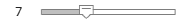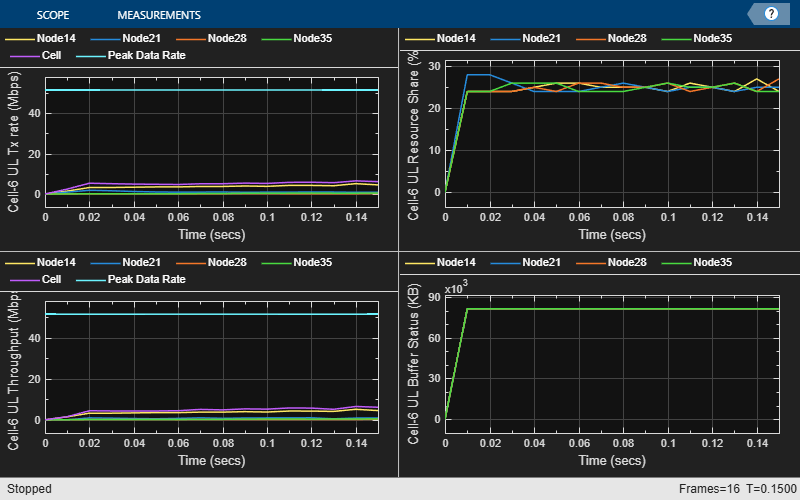# NR Interference Modeling with Toroidal Wrap-Around

This example shows how to model a 19-site cluster with toroidal wrap-around, as described in ITU-R M.2101-0. This example uses the system-level channel model that is specified in 3GPP TR 38.901. The wrap-around provides uniform interference at the cluster edge. All the cells in the cluster operate in the same frequency band with the serving gNB at the center of the cell. You can enable or disable the wrap-around to observe that with wrap-around, the performance metrics of an edge cell become similar to the center cell.

### Introduction

This example models:

• A 19-site cluster with three cells/site, giving a total of 57 cells. A site is represented by 3 colocated gNBs with directional antennas covering 120 degrees area (that is, 3 sectors per site).

• Co-channel intercell interference with wrap-around modeling for removing edge effects.

• System-level channel model based on 3GPP TR 38.901.

• Downlink shared channel (DL-SCH) data transmission and reception.

• DL channel quality measurement by UEs, based on the CSI-RS received from the gNB.

• Uplink shared channel (UL-SCH) data transmission and reception.

• UL channel quality measurement by gNBs, based on the SRS received from the UEs.

Nodes send the control packets (buffer status report (BSR), DL assignment, UL grants, PDSCH feedback, and CSI report) out of band, without the need of resources for transmission and assured error-free reception.

### Toroidal Wrap-around Modeling

To simulate the behavior of a cellular network without introducing edge effects, this example models an infinite cellular network by using toroidal wrap-around. The entire network region relevant for simulations is a cluster of 19 sites (shown bold in this figure). The left-hand figure shows the network region of 19 sites without wrap-around. Site 0 of the central cluster, shown in red, is uniformly surrounded and experiences interference from all sides. A cell in an edge site like site 15 experiences less interference. In the right-hand figure, the wrap-around repeats the original cluster six times to uniformly surround the central cluster.In the wrap-around model, the signal or interference from any UE to a cell is treated as if that UE is in the original cell cluster and the gNB in any of the seven clusters as specified in ITU-R M.2101-0. The distances used to compute the path loss from a transmitter node at $\left(a,b\right)$ to a receiver node at $\left(x,y\right)$ is the minimum of these seven distances.

• Distance between $\left(x,y\right)$ and $\left(a,b\right)$

• Distance between $\left(x,y\right)$ and $\left(a-\sqrt{3}D,b-4D\right)$, where $D$ is the distance between two adjacent gNBs (inter-site distance)

• Distance between $\left(x,y\right)$ and $\left(a+\sqrt{3}D,b+4D\right)$

• Distance between $\left(x,y\right)$ and $\left(a-\frac{3\sqrt{3}}{2}D,b+\frac{7}{2}D\right)$

• Distance between $\left(x,y\right)$ and $\left(a+\frac{3\sqrt{3}}{2}D,b-\frac{7}{2}D\right)$

• Distance between $\left(x,y\right)$ and $\left(a-\frac{5\sqrt{3}}{2}D,b-\frac{1}{2}D\right)$

• Distance between $\left(x,y\right)$ and $\left(a+\frac{5\sqrt{3}}{2}D,b+\frac{1}{2}D\right)$

These equations are derived from the equations in ITU-R M.2101-0 Attachment 2 to Annex 1. In 3GPP TR 38.901 and in the figure above, the rings of 6 and 12 sites around the central site are orientated differently from the orientations in ITU-R M.2101-0, so modified equations are required.

If you disable wrap-around, then the distance between nodes is the Euclidean distance.

### Scenario Configuration

Check if the Communications Toolbox Wireless Network Simulation Library support package is installed. If the support package is not installed, MATLAB® returns an error with a link to download and install the support package.

`wirelessnetworkSupportPackageCheck`

Create a wireless network simulator.

```rng('default'); % Reset the random number generator numFrameSimulation = 1; % Simulation time in terms of number of 10 ms frames networkSimulator = wirelessNetworkSimulator.init();```

Create cell sites, sectors, and UEs in each cell for the urban macro (UMa) scenario.

```numCellSites =19; % Number of cell sites isd = 500; % Inter-site distance in meters numSectors =3; % Number of sectors for each cell site numUEs = 2; % Number of UEs to drop per cell wrapping =true; % Enable toroidal wrap-around modeling of cells. Set it to false to disable toroidal wrap-around % Create the scenario builder scenario = h38901Scenario(Scenario="UMa",FullBufferTraffic="on",NumCellSites=numCellSites,InterSiteDistance=isd,NumSectors=numSectors,NumUEs=numUEs,Wrapping=wrapping); % Build the scenario, adding GNB and UE nodes to the simulator configureSimulator(scenario,networkSimulator);```

Create the system-level channel model for the scenario, and connect it to the simulator.

```% Create the channel model channel = h38901Channel(Scenario="UMa"); % Connect the simulator and channel model connect(channel,networkSimulator,scenario.CellSites);```

#### Logging and Visualization Configuration

Set the `enableTraces` to `true` to log the traces. If the `enableTraces` is set to `false`, then the simulation does not log traces. To speed up the simulation, set it to `false`.

`enableTraces = true;`

Set up scheduling logger and phy logger for the cells of interest.

```% Select the cells (that is, the sites and sectors) for which traces and metrics have to be collected siteOfInterest = [0 15]; sectorOfInterest = [2 2]; numCellsOfInterest = length(siteOfInterest); if enableTraces simSchedulingLogger = cell(numCellsOfInterest,1); simPhyLogger = cell(numCellsOfInterest,1); for cellIdx = 1:numCellsOfInterest % Get the gNB and UEs for the cell of interest from the scenario object [gNB,UEs] = getNodesForCell(scenario,siteOfInterest,sectorOfInterest,cellIdx); % Create an object for scheduler traces logging simSchedulingLogger{cellIdx} = helperNRSchedulingLogger(numFrameSimulation,gNB,UEs); % Create an object for PHY traces logging simPhyLogger{cellIdx} = helperNRPhyLogger(numFrameSimulation,gNB,UEs); end end```

The example updates the metrics plots periodically. Set the number of updates during the simulation.

`numMetricsSteps = 5;`

Set up metric visualizers.

```metricsVisualizer = cell(numCellsOfInterest,1); for cellIdx = 1:numCellsOfInterest % Get the gNB and UEs for the cell of interest from the scenario object [gNB,UEs] = getNodesForCell(scenario,siteOfInterest,sectorOfInterest,cellIdx); % Create visualization object for MAC and PHY metrics metricsVisualizer{cellIdx} = helperNRMetricsVisualizer(gNB,UEs,NumMetricsSteps=numMetricsSteps,PlotSchedulerMetrics=true,PlotPhyMetrics=true,CellOfInterest=siteOfInterest(cellIdx)); end ```

Write the logs to MAT-files. You can use these logs for post-simulation analysis.

`simulationLogFile = "simulationLogs";`

### Simulation

Run the simulation for the specified `numFrameSimulation` frames.

```% Calculate the simulation duration (in seconds) simulationTime = numFrameSimulation * 1e-2; % Run the simulation run(networkSimulator,simulationTime);```### Simulation Visualization

For the cells of interest, run time visualizations show various performance indicators at multiple time steps during the simulation. For a detailed description, see the NR Cell Performance Evaluation with MIMO example.

At the end of the simulation, the achieved values for system performance indicators are compared to their theoretical peak values (considering zero overheads). Performance indicators displayed are achieved data rate (UL and DL), achieved spectral efficiency (UL and DL), and block error rate (BLER) observed for UEs (UL and DL). The peak values are calculated as per 3GPP TR 37.910.

Note that to get meaningful results, the simulation has to be run for a longer duration and for a larger number of UEs per cell.

```for cellIdx = 1:numCellsOfInterest fprintf('\n\nMetrics for site %d, sector %d :\n\n',siteOfInterest(cellIdx),sectorOfInterest(cellIdx)); displayPerformanceIndicators(metricsVisualizer{cellIdx}); end```
```Metrics for site 0, sector 2 : ```
```Peak UL Throughput: 54.64 Mbps. Achieved Cell UL Throughput: 0.81 Mbps Achieved UL Throughput for each UE: [0.4 0.4] Peak UL spectral efficiency: 2.73 bits/s/Hz. Achieved UL spectral efficiency for cell: 0.04 bits/s/Hz Block error rate for each UE in the uplink direction: [0 0] Peak DL Throughput: 75.37 Mbps. Achieved Cell DL Throughput: 9.27 Mbps Achieved DL Throughput for each UE: [0 9.27] Peak DL spectral efficiency: 3.77 bits/s/Hz. Achieved DL spectral efficiency for cell: 0.46 bits/s/Hz Block error rate for each UE in the downlink direction: [1 0] ```
```Metrics for site 15, sector 2 : ```
```Peak UL Throughput: 54.64 Mbps. Achieved Cell UL Throughput: 0.00 Mbps Achieved UL Throughput for each UE: [0 0] Peak UL spectral efficiency: 2.73 bits/s/Hz. Achieved UL spectral efficiency for cell: 0.00 bits/s/Hz Block error rate for each UE in the uplink direction: [1 1] Peak DL Throughput: 75.37 Mbps. Achieved Cell DL Throughput: 10.04 Mbps Achieved DL Throughput for each UE: [0 10.04] Peak DL spectral efficiency: 3.77 bits/s/Hz. Achieved DL spectral efficiency for cell: 0.50 bits/s/Hz Block error rate for each UE in the downlink direction: [1 0] ```

### Simulation Logs

Save the simulation logs related to cells of interest into a MAT file. For a description of the simulation logs format, see the NR Cell Performance Evaluation with MIMO example.

```if enableTraces simulationLogs = cell(numCellsOfInterest,1); for cellIdx = 1:numCellsOfInterest % Get the gNB for the cell of interest from the scenario object gNB = getNodesForCell(scenario,siteOfInterest,sectorOfInterest,cellIdx); if gNB.DuplexMode == "FDD" logInfo = struct('DLTimeStepLogs',[],'ULTimeStepLogs',[],... 'SchedulingAssignmentLogs',[],'PhyReceptionLogs',[]); [logInfo.DLTimeStepLogs,logInfo.ULTimeStepLogs] = getSchedulingLogs(simSchedulingLogger{cellIdx}); else % TDD logInfo = struct('TimeStepLogs',[],'SchedulingAssignmentLogs',[],'PhyReceptionLogs',[]); logInfo.TimeStepLogs = getSchedulingLogs(simSchedulingLogger{cellIdx}); end % Get the scheduling assignments log logInfo.SchedulingAssignmentLogs = getGrantLogs(simSchedulingLogger{cellIdx}); % Get the phy reception logs logInfo.PhyReceptionLogs = getReceptionLogs(simPhyLogger{cellIdx}); simulationLogs{cellIdx} = logInfo; end % Save simulation logs in a MAT-file save(simulationLogFile,'simulationLogs'); end```

### Local Functions

```function [gNB,UEs] = getNodesForCell(scenario,siteOfInterest,sectorOfInterest,cellIdx) siteIdx = siteOfInterest(cellIdx) + 1; sectorIdx = sectorOfInterest(cellIdx) + 1; numCellSites = numel(scenario.CellSites); if (siteIdx > numCellSites) warning('The cell site of interest (%d) does not exist, using the last cell site (%d).',siteIdx-1,numCellSites-1); siteIdx = numCellSites; end numSectors = numel(scenario.CellSites(siteIdx).Sectors); if (sectorIdx > numSectors) warning('For cell site %d, the sector of interest (%d) does not exist, using the last sector (%d).',siteIdx-1,sectorIdx-1,numSectors-1); sectorIdx = numSectors; end gNB = scenario.CellSites(siteIdx).Sectors(sectorIdx).BS; UEs = scenario.CellSites(siteIdx).Sectors(sectorIdx).UEs; end```

 3GPP TS 38.104. “NR; Base Station (BS) radio transmission and reception.” 3rd Generation Partnership Project; Technical Specification Group Radio Access Network.

 3GPP TS 38.214. “NR; Physical layer procedures for data.” 3rd Generation Partnership Project; Technical Specification Group Radio Access Network.

 3GPP TS 38.321. “NR; Medium Access Control (MAC) protocol specification.” 3rd Generation Partnership Project; Technical Specification Group Radio Access Network.

 3GPP TS 38.322. “NR; Radio Link Control (RLC) protocol specification.” 3rd Generation Partnership Project; Technical Specification Group Radio Access Network.

 3GPP TS 38.323. “NR; Packet Data Convergence Protocol (PDCP) specification.” 3rd Generation Partnership Project; Technical Specification Group Radio Access Network.

 3GPP TS 38.331. “NR; Radio Resource Control (RRC) protocol specification.” 3rd Generation Partnership Project; Technical Specification Group Radio Access Network.

 3GPP TR 37.910. “Study on self evaluation towards IMT-2020 submission.” 3rd Generation Partnership Project; Technical Specification Group Radio Access Network.